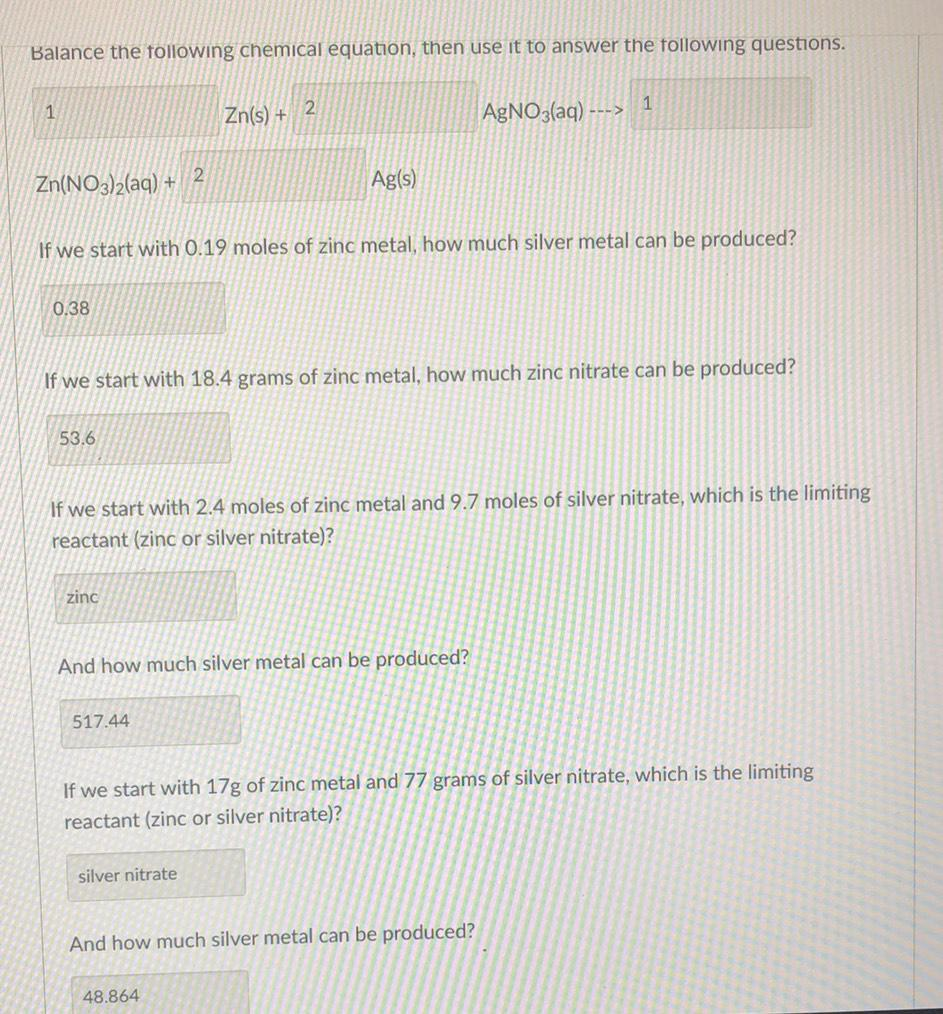Question:

# Balance the following chemical equation, then use it to answer the following questions. 1 1 2. Zn(s) + AgNO3(aq) ---> Zn(NO3)2(aBalance the following chemical equation, then use it to answer the following questions. 1 1 2. Zn(s) + AgNO3(aq) ---> Zn(NO3)2(aq) + 2 Ag(s) If we start with 0.19 moles of zinc metal, how much silver metal can be produced? 0.38 If we start with 18.4 grams of zinc metal, how much zinc nitrate can be produced? 53.6 If we start with 2.4 moles of zinc metal and 9.7 moles of silver nitrate, which is the limiting reactant (zinc or silver nitrate)? zinc And how much silver metal can be produced? 517.44 If we start with 17g of zinc metal and 77 grams of silver nitrate, which is the limiting reactant (zinc or silver nitrate)? silver nitrate And how much silver metal can be produced? 48.864